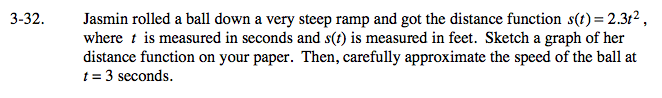### Home > CALC > Chapter 3 > Lesson 3.1.2 > Problem3-32

3-32.The velocity/speed at t = 3 should be the same as the IROC (or slope of the tangent line) at t = 3.

v(3) = 13.8 ____________ don't forget units!

Find the slope function of s(t) by finding the IROC at x = 3:

$\text{IROC}_{t=3}=\lim_{h\rightarrow 0}\frac{f(3+h)-f(3)}{h}$

1. Evaluate when f(x) = s(t) = 2.3t3.
2. Expand and simplify the numerator.
3. Factor out an h from the numerator.
4. 'Cancel out' the h from the fraction.

$5.\text{ Evaluate the }\lim_{h\rightarrow 0}.\text{ This is the velocity at }t=3.$

v(3) can also be found with Power Rule:
s(t) = 2.3t2
v(t) = 4.6t
v(3) = _____________

Use the eTool below to help solve the problem.
Click on the link to the right to view the full version of the eTool. Calc 3-32 HW eTool